# Garden plot

Calculate how many meters of fence need to fence the square garden with length and width of 22 meters.

Result

x =  88 m

#### Solution:Leave us a comment of example and its solution (i.e. if it is still somewhat unclear...):Be the first to comment!## Next similar examples:

1. Fence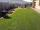Square garden has an area of 537 m2. How many meters netting is necessary to fence the garden?
2. A rectangularA rectangular garden 40 m long and 30 m wide is to be fenced with fence posts at each corner. All the other posts will be 5 meters a part. How many posts will be needed to fence in the garden.
3. Area of squareCalculate the content area of the square whose perimeter is 24 dm.
4. RectangleCalculate perimeter of the rectangle with sides a=2.4 m and b=1.9 m.
5. DoctorsIn the city operates 171 doctors. The city has 128934 citizens. How many citizens are per one doctor?
6. Rectangle vs square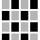The rectangle has dimensions of 13 × 10, square 8 × 8. Which shape has more area and how much above?
7. Perimeter from areaWhat is the perimeter of the square if its content is 64 cm2?
8. Unknown number 24I think the number: a - is the same as the square area that has the 12th circumfence. What is this number? b - its half is 7 times bigger than its quarter. Is this the number?
9. Compare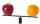Compare with characters >, <, =: 85.57 ? 80.83
10. To thousands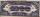Round to thousands following numbers:
11. ProductResult of the product of the numbers 1, 2, 3, 1, 2, 0 is:
12. Roman numerals 2+Add up the number writtens in Roman numerals. Write the results as a roman numbers.Added together and write as decimal number: LXVII + MLXIVWrite numbers written in Roman numerals as decimal.Add up the number writtens in Roman numerals. Write the results as a decimal number.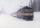The train passes part of the line for 95 minutes at speed 75 km/h. What speed would have to go in order to shorten the driving time of 20 minutes?Prove whether you can construct a triangle ABC, if a=9 cm, b=10 cm, c=4 cm.